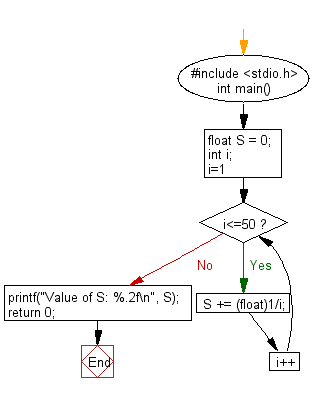﻿ C : Calculate the value of a specified series of 50 numbers

# C Exercises: Calculate the value of a specified series of 50 numbers

## C Basic Declarations and Expressions: Exercise-45 with Solution

Write a C program to calculate the value of S where S = 1 + 1/2 + 1/3 + … + 1/50.

Sample Solution:

C Code:

``````#include <stdio.h>
int main() {
float S = 0;
int i;

// Loop to calculate the sum
for(i=1; i<=50; i++) {
S += (float)1/i;
}

// Print the result
printf("Value of S: %.2f\n", S);

return 0;
}
``````

Sample Output:

```Value of S: 4.50
```

Flowchart:C programming Code Editor:

What is the difficulty level of this exercise?

Test your Programming skills with w3resource's quiz.

﻿